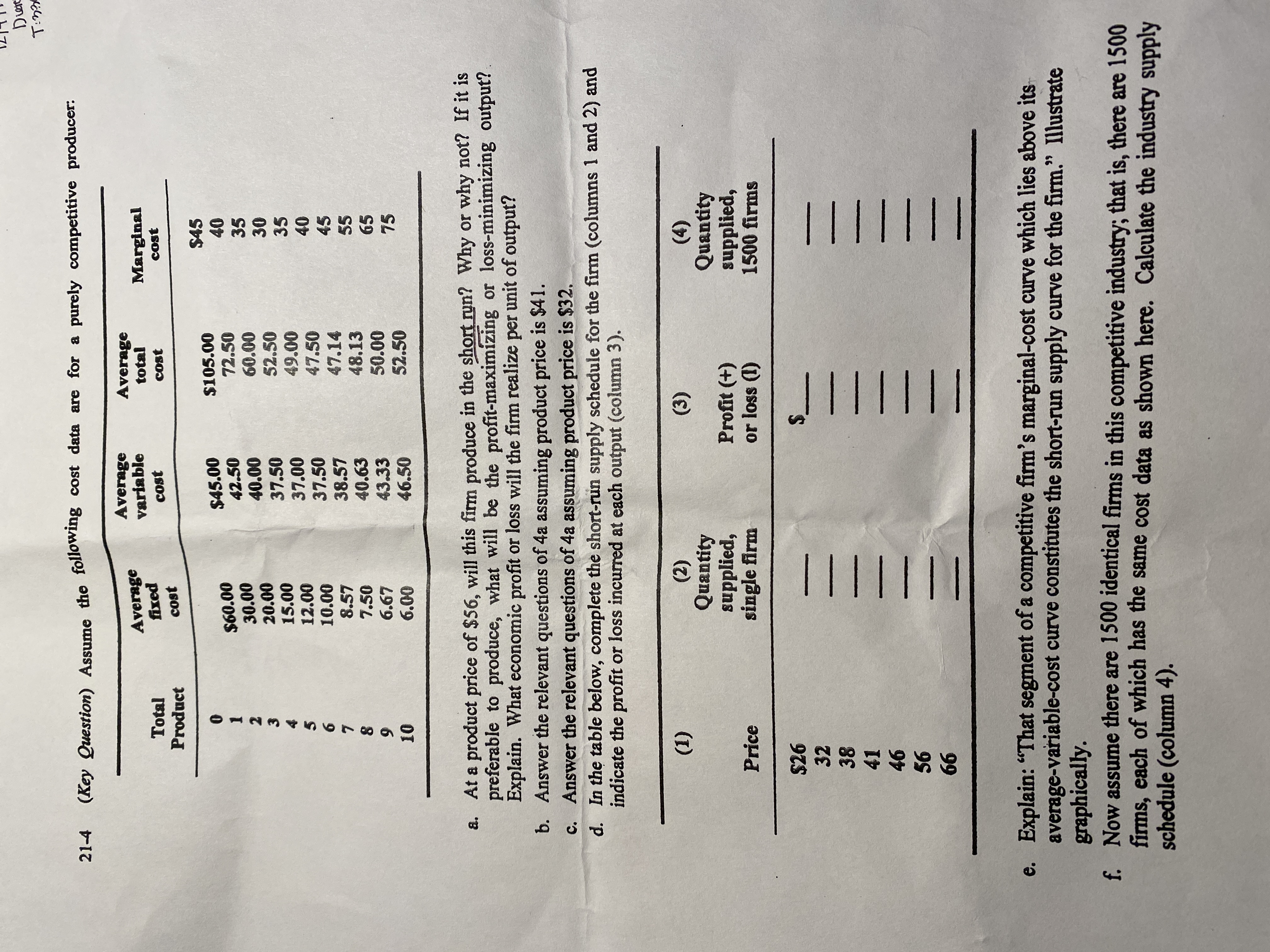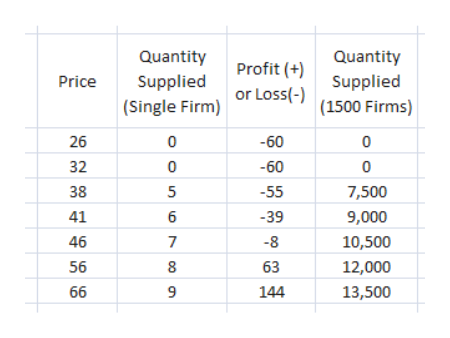# DereT:n?(Key Question) Assume the following cost data are for a purely competitive producer:21-4AveragevariableAveragetotalAveragefixedMarginalcostTotalProductcostcostcost\$4540\$105.0072.5060.0052.5049.0047.50\$45.0042.5040.00\$60.0030.0020.0015.0012.0010.00353037.5037.0037.5038.5740.6343.3346.503540456.47.14558.5748.1350.0052.50657.506.6775106.00a. At a product price of \$56, will this firm produce in the short run? Why or why not? If it ispreferable to produce, what will be the profit-maximízing or loss-minimizing output?Explain. What economic profit or loss will the firm realize per unit of output?b. Answer the relevant questions of 4a assuming product price is \$41.c. Answer the relevant questions of 4a assuming product price is \$32.d. In the table below, complete the short-run supply schedule for the firm (columns 1 and 2) andindicate the profit or loss incurred at each output (column 3).(4)Quantitysupplied,1500 firms(3)(2)Quantitysupplied,single firm(1)Profit (+)or loss (1)Price\$26323841465666e. Explain: "That segment of a competitive firm's marginal-cost curve which lies above itsaverage-variable-cost curve constitutes the short-run supply curve for the firm." Illustrategraphically.f. Now assume there are 1500 identical firms in this competitive industry; that is, there are 1500firms, each of which has the same cost data as shown here. Calculate the industry supplyschedule (column 4).99TI||||JIII|||TI||||

Question
14 views

Hello, can I get help with the last three parts of this question?help_outlineImage TranscriptioncloseDere T:n? (Key Question) Assume the following cost data are for a purely competitive producer: 21-4 Average variable Average total Average fixed Marginal cost Total Product cost cost cost \$45 40 \$105.00 72.50 60.00 52.50 49.00 47.50 \$45.00 42.50 40.00 \$60.00 30.00 20.00 15.00 12.00 10.00 35 30 37.50 37.00 37.50 38.57 40.63 43.33 46.50 35 40 45 6. 47.14 55 8.57 48.13 50.00 52.50 65 7.50 6.67 75 10 6.00 a. At a product price of \$56, will this firm produce in the short run? Why or why not? If it is preferable to produce, what will be the profit-maximízing or loss-minimizing output? Explain. What economic profit or loss will the firm realize per unit of output? b. Answer the relevant questions of 4a assuming product price is \$41. c. Answer the relevant questions of 4a assuming product price is \$32. d. In the table below, complete the short-run supply schedule for the firm (columns 1 and 2) and indicate the profit or loss incurred at each output (column 3). (4) Quantity supplied, 1500 firms (3) (2) Quantity supplied, single firm (1) Profit (+) or loss (1) Price \$26 32 38 41 46 56 66 e. Explain: "That segment of a competitive firm's marginal-cost curve which lies above its average-variable-cost curve constitutes the short-run supply curve for the firm." Illustrate graphically. f. Now assume there are 1500 identical firms in this competitive industry; that is, there are 1500 firms, each of which has the same cost data as shown here. Calculate the industry supply schedule (column 4). 99 TI|||| JIII||| TI|||| fullscreen
check_circle

star
star
star
star
star
1 Rating
Step 1

d) For price equal to 26 and 32 dollars there is no AVC less than P thus, quantity supplied is zero. At price equal to 38 P is greater than AVC at Q = 5 and Q = 4 units. The loss at Q = 4 is of 58 dollars which is higher than the loss at Q =5 of 55 dollars. Thus, at P = 38, Q = 5. At further prices quantity supplied is shown in the table below.

Loss when quantity supplied is zero is of fixed cost which is 60 dollars.

Profit/Loss when some unit of goods are supplied is calculated by subtracting Total cost (TC) from Total revenue (TR).

TC = ATC * Q

TR = P*Qhelp_outlineImage TranscriptioncloseQuantity Quantity Profit (+) Price Supplied Supplied or Loss(-) (Single Firm) |(1500 Firms) -60 26 -60 32 38 -55 7,500 41 6 -39 9,000 46 -8 10,500 63 56 12,000 66 144 13,500 fullscreen
Step 2

e) When price is less than AVC, the firm is not able to cover up its both fixed cost and a part of it variable cost and thus, it is better for a firm to stop producing till the point P < AVC. Thus, short run equilibrium output is zero when P is less than AVC.

Now at a price OP1 P = MC = minimum of AVC such that a firm is just able to cover its variable cost and it does not cover u...

### Want to see the full answer?

See Solution

#### Want to see this answer and more?

Solutions are written by subject experts who are available 24/7. Questions are typically answered within 1 hour.*

See Solution
*Response times may vary by subject and question.
Tagged in